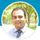cancel
Showing results for
Did you mean:Anonymous
Not applicable

## Count multiple specific numbers of values in a row

Hey guys

I'm quit new in DAX and i'm working on a report which I have no idea how to solve. Its the following problem:What do I need?

I want to have an extra colum with the following rule:

Yes: If the colums Budget, Fortschritt, Risiken, Ressourcen and Termin contain one or more of the number "3" AND three or more of the number 2. So if either of them applies, it should give out a "yes"

No: if the rule above does not apply

The rows which are marked yellow in the example should have a yes, and the last one should have a now.

Thanks for the help!

1 ACCEPTED SOLUTIONSuper User

@Anonymous ,

Try a new column like

switch ( True(),
[Budget] >=3 || [Fortschritt]>=3 || [Risiken]>=3 || [Ressourcen]>=3 || [Termin]>=3 , "Yes",
if([Budget]=2,1,0) + if([Fortschritt]=2,1,0) + if([Risiken]=2,1,0) + if([Ressourcen]=2,1,0) + if([Termin]=2,1,0) >=3, "Yes",
"No"
)

3 REPLIES 3Super User

@Anonymous ,

Try a new column like

switch ( True(),
[Budget] >=3 || [Fortschritt]>=3 || [Risiken]>=3 || [Ressourcen]>=3 || [Termin]>=3 , "Yes",
if([Budget]=2,1,0) + if([Fortschritt]=2,1,0) + if([Risiken]=2,1,0) + if([Ressourcen]=2,1,0) + if([Termin]=2,1,0) >=3, "Yes",
"No"
)Anonymous
Not applicable

Thanks @amitchandak  for the quick answer. I tested it and it works perfectly!!Community Champion

Hi @Anonymous

Try this as a Calculated Column

``````New Column =
SWITCH (
TRUE (),
Table[Budget]
|| Table[Fortschritt]
|| Table[Risiken]
|| Table[Ressourcen]
|| Table[Termin] = 3, "Yes",
Table[Budget] + Table[Fortschritt] + Table[Risiken] + Table[Ressourcen] + Table[Termin] >= 8, "Yes",
"No"
)
``````

Regards,
Harsh Nathani
Did I answer your question? Mark my post as a solution! Appreciate with a Kudos!! (Click the Thumbs Up Button)